##账号 自动登录 找回密码 密码 立即注册
 搜索

# 成都趣题 第500001期 十点半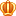发表于 2023-1-8 14:15:25 | 显示全部楼层 |阅读模式

[/hide]

 名次 第1名 第2名 第3名 第4名 第5名 谁 等待着变成王者登上宝座~ 语言 几楼 奖励 展示+8荣誉7鱼币2贡献1技术值最佳答案 展示+4荣誉4鱼币 展示+3荣誉3鱼币 展示+2荣誉 展示

 1.  5星 80.00% (4) 2.  4星 20.00% (1) 3.  3星 0.00% (0) 4.  2星 0.00% (0) 5.  1星 0.00% (0) 您所在的用户组没有投票权限发表于 2023-1-8 16:10:06 | 显示全部楼层
 本帖最后由 sfqxx 于 2023-1-8 16:14 编辑 @hveagle 在哪玩？怎么玩才能获得奖励？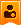楼主| 发表于 2023-1-8 16:15:11 | 显示全部楼层
 sfqxx 发表于 2023-1-8 16:10 @hveagle 在哪玩？怎么玩才能获得奖励？ 发趣题发表于 2023-1-8 16:16:52 | 显示全部楼层
 哦发表于 2023-1-8 16:17:07 | 显示全部楼层
 那我要完了 我不会楼主| 发表于 2023-1-8 16:18:28 | 显示全部楼层
 sfqxx 发表于 2023-1-8 16:17 那我要完了 我不会 你发Scratch发表于 2023-1-8 16:19:08 | 显示全部楼层
 代码怎么发（除图片）发表于 2023-1-8 16:19:39 | 显示全部楼层
 它是一块一块的发表于 2023-1-8 18:40:05 | 显示全部楼层
 然后我要做什么？楼主| 发表于 2023-1-8 19:08:21 | 显示全部楼层
 zhangjinxuan 发表于 2023-1-8 18:40 然后我要做什么？ 你报名了吗？发表于 2023-1-8 19:42:03 | 显示全部楼层
 没看懂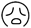发表于 2023-1-8 21:05:25 | 显示全部楼层
 本帖最后由 AhrimanSefid 于 2023-1-8 21:12 编辑 import random cards = {"A":1,"2":2,"3":3,"4":4,"5":5,"6":6,"7":7,"8":8,"9":9,"J":0.5,"Q":0.5,"K":0.5,"Little Joker":0.5,"Big Joker":0.5} player = True player_card = [] player_card_number = [] computer = True computer_card = [] computer_card_number = [] print("目前有这些卡牌：") for item in cards.keys():         print(item) for i in range(5):         player_card.append(random.sample(cards.keys,1))         player_card_number.append(player_card[i])         computer_card.append(random.sample(cards.keys,1))         computer_card_number.append(computer_card[i]) player_number_total = player_card_number+player_card_number+player_card_number+player_card_number+player_card_number computer_number_total = computer_card_number+computer_card_number+computer_card_number+computer_card_number+computer_card_number print("玩家的牌是：",player_card) print("玩家总共有",str(player_number_total),"点") print("电脑的牌是：",computer_card) print("电脑总共有",str(computer_number_total),"点") if player_number_total > 10.5:         print("你被淘汰了哟")         player = False else:         pass if computer_number_total > 10.5:         print("电脑被淘汰了")         computer = False else:         pass if player == True and computer == True:         print("你们还可以继续游戏")         if player_number_total != 10.5 and computer_number_total != 10.5:                 player_ask_number = int(input("你想要再抽几张牌？(1~4)"))                 computer_ask_number = random.randint(1,4)                 for i in range(player_ask_number):                         player_card.append(random.sample(cards.keys,1))                         player_card_number.append(player_card[i+5])                 for i in range(computer_ask_number):                         computer_card.append(random.sample(cards.keys,1))                         computer_card_number.append(computer_card[i+5])                 player_number_len = len(player_card_number)                 computer_number_len = len(computer_card_number)                 player_number_total = player_card_number+player_card_number+player_card_number+player_card_number+player_card_number+player_card_number+player_card_number[player_number_len]                 computer_number_total = computer_card_number+computer_card_number+computer_card_number+computer_card_number+computer_card_number+computer_card_number+computer_card_number[computer_number_len]                 if player_number_total > 10.5 and computer_number_total > 10.5:                         print("都没有赢哟，再来一局吧")                 elif player_number_total <= 10.5 and computer_number_total > 10.5:                         print("哈，你赢了，祝贺")                 elif player_number_total > 10.5 and computer_number_total <= 10.5:                         print("电脑赢了，继续加油")                 else:                         if player_number_total > computer_number_total:                                 print("哈，你赢了，祝贺")                         elif player_number_total < computer_number_total:                                 print("电脑赢了，继续加油")                         else:                                 print("平局哟，再来一局吧")         elif player_number_total == 10.5 and computer_number_total != 10.5:                 print("哈，你赢了，祝贺")         elif player_number_total != 10.5 and computer_number_total == 10.5:                 print("电脑赢了，继续加油")         else:                 print("都没有赢哟，再来一局吧") elif player == True and computer == False:         print("哈，你赢了，祝贺") elif player == False and computer == True:         print("电脑赢了，继续加油") else:         print("都没有赢哟，再来一局吧")复制代码 耗时1.5小时，干就完了 PS:由于是直接写，没有调试，可能会报错，后面我会修改的发表于 2023-1-8 21:13:57 | 显示全部楼层
 AhrimanSefid 发表于 2023-1-8 21:05 耗时1.5小时，干就完了 PS:由于是直接写，没有调试，可能会报错，后面我会修改的 修改：TypeError: Population must be a sequence.  For dicts or sets, use sorted(d). import random cards = {"A":1,"2":2,"3":3,"4":4,"5":5,"6":6,"7":7,"8":8,"9":9,"J":0.5,"Q":0.5,"K":0.5,"Little Joker":0.5,"Big Joker":0.5} player = True player_card = [] player_card_number = [] computer = True computer_card = [] computer_card_number = [] print("目前有这些卡牌：") for item in cards.keys():         print(item) for i in range(5):         player_card.append(random.sample(list(cards.keys),1))         player_card_number.append(player_card[i])         computer_card.append(random.sample(list(cards.keys),1))         computer_card_number.append(computer_card[i]) player_number_total = player_card_number+player_card_number+player_card_number+player_card_number+player_card_number computer_number_total = computer_card_number+computer_card_number+computer_card_number+computer_card_number+computer_card_number print("玩家的牌是：",player_card) print("玩家总共有",str(player_number_total),"点") print("电脑的牌是：",computer_card) print("电脑总共有",str(computer_number_total),"点") if player_number_total > 10.5:         print("你被淘汰了哟")         player = False else:         pass if computer_number_total > 10.5:         print("电脑被淘汰了")         computer = False else:         pass if player == True and computer == True:         print("你们还可以继续游戏")         if player_number_total != 10.5 and computer_number_total != 10.5:                 player_ask_number = int(input("你想要再抽几张牌？(1~4)"))                 computer_ask_number = random.randint(1,4)                 for i in range(player_ask_number):                         player_card.append(random.sample(list(cards.keys),1))                         player_card_number.append(player_card[i+5])                 for i in range(computer_ask_number):                         computer_card.append(random.sample(list(cards.keys),1))                         computer_card_number.append(computer_card[i+5])                 player_number_len = len(player_card_number)                 computer_number_len = len(computer_card_number)                 player_number_total = player_card_number+player_card_number+player_card_number+player_card_number+player_card_number+player_card_number+player_card_number[player_number_len]                 computer_number_total = computer_card_number+computer_card_number+computer_card_number+computer_card_number+computer_card_number+computer_card_number+computer_card_number[computer_number_len]                 if player_number_total > 10.5 and computer_number_total > 10.5:                         print("都没有赢哟，再来一局吧")                 elif player_number_total <= 10.5 and computer_number_total > 10.5:                         print("哈，你赢了，祝贺")                 elif player_number_total > 10.5 and computer_number_total <= 10.5:                         print("电脑赢了，继续加油")                 else:                         if player_number_total > computer_number_total:                                 print("哈，你赢了，祝贺")                         elif player_number_total < computer_number_total:                                 print("电脑赢了，继续加油")                         else:                                 print("平局哟，再来一局吧")         elif player_number_total == 10.5 and computer_number_total != 10.5:                 print("哈，你赢了，祝贺")         elif player_number_total != 10.5 and computer_number_total == 10.5:                 print("电脑赢了，继续加油")         else:                 print("都没有赢哟，再来一局吧") elif player == True and computer == False:         print("哈，你赢了，祝贺") elif player == False and computer == True:         print("电脑赢了，继续加油") else:         print("都没有赢哟，再来一局吧") 复制代码发表于 2023-1-9 14:42:11 | 显示全部楼层
 AhrimanSefid 发表于 2023-1-8 21:13 修改：TypeError: Population must be a sequence.  For dicts or sets, use sorted(d). Traceback (most recent call last):   File "C:\Users\Administrator\Desktop\Python\test\t1.py", line 18, in     player_card.append(random.sample(list(cards.keys),1)) TypeError: 'builtin_function_or_method' object is not iterable发表于 2023-1-9 16:05:49 | 显示全部楼层
 chinajz 发表于 2023-1-9 14:42 Traceback (most recent call last):   File "C:%users\Administrator\Desktop\Python\test\t1.py", lin ... 这个bug我也发现了，但是我在网上查了一堆资料，都没发现我哪里错了，网上大多数都是说这是由于python的内置函数忘了加括号，但我是检查了n遍，就是没发现哪里没有括号，有大佬会的能不能帮我修改一下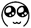发表于 2023-1-10 18:09:36 | 显示全部楼层
 AhrimanSefid 发表于 2023-1-9 16:05 这个bug我也发现了，但是我在网上查了一堆资料，都没发现我哪里错了，网上大多数都是说这是由于python的 ... keys后面没加括号发表于 2023-1-10 20:13:22 | 显示全部楼层
 tommyyu 发表于 2023-1-10 18:09 keys后面没加括号 感谢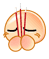修改后的代码： import random cards = {"A":1,"2":2,"3":3,"4":4,"5":5,"6":6,"7":7,"8":8,"9":9,"J":0.5,"Q":0.5,"K":0.5,"Little Joker":0.5,"Big Joker":0.5} player = True player_card = [] player_card_number = [] computer = True computer_card = [] computer_card_number = [] print("目前有这些卡牌：") for item in cards.keys():         print(item) for i in range(5):         player_card.append(random.sample(list(cards.keys()),1))         player_card_number.append(player_card[i])         computer_card.append(random.sample(list(cards.keys()),1))         computer_card_number.append(computer_card[i]) player_number_total = player_card_number+player_card_number+player_card_number+player_card_number+player_card_number computer_number_total = computer_card_number+computer_card_number+computer_card_number+computer_card_number+computer_card_number print("玩家的牌是：",player_card) print("玩家总共有",str(player_number_total),"点") print("电脑的牌是：",computer_card) print("电脑总共有",str(computer_number_total),"点") if player_number_total > 10.5:         print("你被淘汰了哟")         player = False else:         pass if computer_number_total > 10.5:         print("电脑被淘汰了")         computer = False else:         pass if player == True and computer == True:         print("你们还可以继续游戏")         if player_number_total != 10.5 and computer_number_total != 10.5:                 player_ask_number = int(input("你想要再抽几张牌？(1~4)"))                 computer_ask_number = random.randint(1,4)                 for i in range(player_ask_number):                         player_card.append(random.sample(list(cards.keys()),1))                         player_card_number.append(player_card[i+5])                 for i in range(computer_ask_number):                         computer_card.append(random.sample(list(cards.keys()),1))                         computer_card_number.append(computer_card[i+5])                 player_number_len = len(player_card_number)                 computer_number_len = len(computer_card_number)                 player_number_total = player_card_number+player_card_number+player_card_number+player_card_number+player_card_number+player_card_number+player_card_number[player_number_len]                 computer_number_total = computer_card_number+computer_card_number+computer_card_number+computer_card_number+computer_card_number+computer_card_number+computer_card_number[computer_number_len]                 if player_number_total > 10.5 and computer_number_total > 10.5:                         print("都没有赢哟，再来一局吧")                 elif player_number_total <= 10.5 and computer_number_total > 10.5:                         print("哈，你赢了，祝贺")                 elif player_number_total > 10.5 and computer_number_total <= 10.5:                         print("电脑赢了，继续加油")                 else:                         if player_number_total > computer_number_total:                                 print("哈，你赢了，祝贺")                         elif player_number_total < computer_number_total:                                 print("电脑赢了，继续加油")                         else:                                 print("平局哟，再来一局吧")         elif player_number_total == 10.5 and computer_number_total != 10.5:                 print("哈，你赢了，祝贺")         elif player_number_total != 10.5 and computer_number_total == 10.5:                 print("电脑赢了，继续加油")         else:                 print("都没有赢哟，再来一局吧") elif player == True and computer == False:         print("哈，你赢了，祝贺") elif player == False and computer == True:         print("电脑赢了，继续加油") else:         print("都没有赢哟，再来一局吧") 复制代码

 您需要登录后才可以回帖 登录 | 立即注册 本版积分规则 回帖后跳转到最后一页